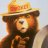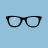# Pivot Significance Index for ThinkorSwim#### markos

##### Well-known member
VIP
Hint: Measures time and price between pivots and uses that data to indicate how meaningful a pivot may be

Trading Notes - the higher the indicator values, the farther the distance between pivots

Both indicator lines at 1 indicate an area is farther than "normal" in both time and price from the most recent pivot. Look for white line as a place for trend to rest

Cyan line at 1 and Orange line at 0 indicate an area where price moved quickly. This "divergence" is the kind dip buyers would want to see

Code:
``````# Pivot Significance Index in 6-3-19 TSL
# v0.01
# 5.19.19
# Nube

# Subscript used later

script normalize{

input h = high;

input l = low;

input c = close;

input x = 21;

def normalize = if Highest(h, x) - Lowest(l, x) != 0

then (c - Lowest(l, x)) / (Highest(h, x) - Lowest(l, x))

else 0;

plot normalized = normalize;

}

# Inputs

input pivotLength = 4; #hint pivotLength: Length for stochastic pivot

input normalizationLength = 21; #hint normalizationLength: Length for normalization of time and price between pivots

# Variables

def h = high;

def l = low;

def c = close;

def nan = Double.NaN;

def x = BarNumber();

def norm = normalize(h, l, c, pivotLength);

## Pivot High

def hh = if norm  crosses above .5

then h

else if h > hh

then h

else hh;

def hpBar = if h == hh

then x

else nan;

def hpBar_1 = if !IsNaN(hpBar)

then x

else hpBar_1;

def hpPrice = if !IsNaN(hpBar)

then h

else hpPrice;

## Pivot Low

def ll = if norm crosses below .5

then l

else if l < ll

then l

else ll;

def lpBar = if l == ll

then x

else nan;

def lpBar_1 =  if !IsNaN(lpBar)

then x

else lpBar_1;

def lpPrice = if !IsNaN(lpBar)

then l

else lpPrice;

### Normalizing distance between pivots

def bars  = hpBar_1 - lpBar_1;

def price = hpPrice - lpPrice;

def nBarsBetween  = normalize(bars, bars, bars, normalizationLength);

def nPriceBetween = normalize(price, price, price, normalizationLength);

# Plots

plot

SignificantPivot = if !IsNaN(c) and nBarsBetween + nPriceBetween == 2 then 1 else nan;

SignificantPivot.SetLineWeight(3);

SignificantPivot.SetDefaultColor(Color.White);

plot

BarsBetweenPivots = if IsNaN(c) then nan else nbarsBetween;

BarsBetweenPivots.SetDefaultColor(Color.Dark_Orange);

plot

PriceBetweenPivots = if IsNaN(c) then nan else nPriceBetween;

PriceBetweenPivots.SetDefaultColor(Color.Cyan);

declare lower;

# f/ Pivot Significance Index``````

Last edited:
J

#### Jabbo

##### New member
2019 Donor
I am having a "invalid statement at my 34:1 -" } " not sure what is going on. Is this statement correct?

Thank you!#### horserider

##### Well-known member
VIP
@Jabbo I am guessing a missing # here: Inputs should be # Inputs#### markos

##### Well-known member
VIP
I placed the code into my editor and didn't see the problem. I then cleaned up some blank lines. @Jabbo please let me know if you still have problems. Markos2nd edit (not SOLVED) How to remove previous days plots (on Pivot Points)? Questions 2Heiken Ashi Pivot "Strategy" - mobius - help question. Questions 2